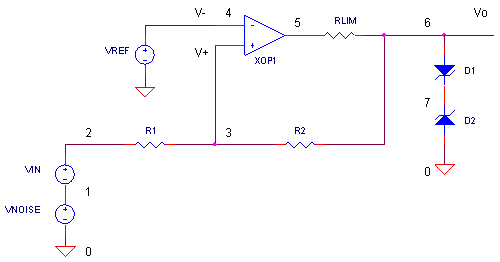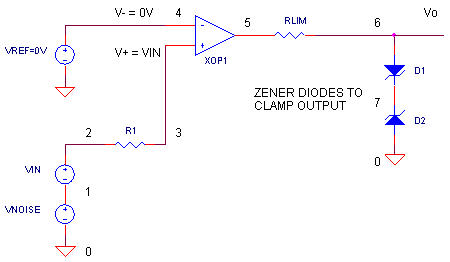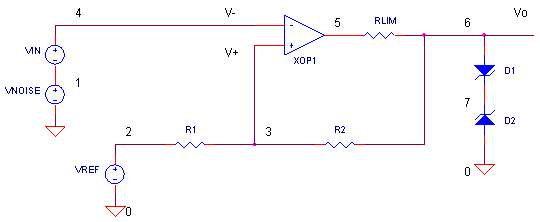eCircuit  Center

### Op Amp Comparator with Hysteresis

CIRCUITThe challenge sounds simple enough - take a 60 Hz (or 50 Hz) sinewave from the AC power line and convert it to a square wave. This signal will serve as a clock to drive counters for a 24 hour time clock. So you hook up an op amp as a comparator to do the job. But your surprised to see the clock running too fast! With oscilloscope in hand you discover the AC line is noisy! And to your horror, you see glitches (additional edges) at the comparator's output, causing the counters to advance too quickly. What you need is a better comparator, immune to the noise swinging above and below the comparator's threshold.

SIMPLE COMPARATOR

The elementary comparator looks similar to circuit below.You connect V- to ground (0V), then apply Vin to V+. (Since there's no current through R1, VIN essentially appears at V+.) What happens at the output? In theory, when Vin > V- = 0V, the output goes to a POSITIVE Output State. And, not surprising, when Vin < V- = 0V, the output goes to a NEGATIVE Output State.  Zener diodes D1 and D2 set the positive and negative output levels: VP = Vf D1 + VZ D2  and VN = Vf D2 + VZ D1.

CIRCUIT INSIGHT   VIN applies a 10V peak sinewave to the input. The output swings to the POSITIVE and NEGATIVE States of +5 V and -5 V, respectively. Run a simulation of OP_COMP.CIR. Plot the input V(2) and output V(6). It appears the comparator performs well as a zero-crossing detector and all is right with the world.

However, the real world throws in a few "goodies" for free - mostly noise where you need it the least. So let's add some noise by changing the VNOISE statement to read 1VPEAK instead of 0VPEAK. VNOISE adds a 1V @ 2.5 kHz sinewave ontop of our pristine 60 Hz. Rerun the simulation and let's look at our output now. Unfortunately, our fine square wave is rife chatter - it no longer provides one rising edge per 60 Hz cycle!

COMPARATOR WITH HYSTERESIS

How do we overcome the problems of the basic comparator? Just add some positive feedback to the circuit.Notice, how V+ is developed - its now a combination of the both the input VIN and the output state Vo. This basically means that there are two thresholds: one when for a POSITIVE Output State and another for the NEGATIVE Output State. (For now, assume VREF = 0)

What are these two thresholds? Some simple math uncovers the answer. First, we need to find the voltage at V+.

V+  =  VIN R2/(R1 + R2)    +    Vo R1/(R1 + R2)

Next, recall that the op amp's switching threshold occurs at V+ = V- = 0. So the question becomes, what value of VIN causes V+ = 0V. To accomplish this, we solve the above equation for VIN when V+ = 0. The only tricky part lies in the fact that one of the variables, Vo, can be in one of two states:

POSITIVE  Output State      Vo = VP
NEGATIVE Output State      Vo = VN

So we solve the above equation first with Vo = VP and then Vo = VN. We get two thresholds for VIN:

Vth+ = - VN R1 / R2
Vth-  = - VP R1 / R2

In a nutshell, this is how the comparator operates.

To get to the POSITIVE Output State
Assume Vo = VN.
The input must rise above the threshold: VIN > Vth+

To get to the NEGATIVE Output State
Assume Vo = VP.
The input must fall below the threshold: VIN < Vth-

Having two thresholds based on both the input VIN and the current output state is called hysteresis. As an example, suppose VP = +5V, VN = -5V, R1 = 1k and R2 = 5k. You get two thresholds

Vth+ = - (-5V) 1k / 5k
= +1V
Vth-  = - 5V 1k / 5k
= -1V

CIRCUIT INSIGHT   Okay, let's see the two thresholds in action. Add R2 into the picture by removing the comment character "*" before the R2 statement. Also return VNOISE to 0VPEAK. Run a new simulation of OP_COMP.CIR and plot the input V(2) and output V(6). Check it out! The output switches at VIN = 1 V on the way up, but, switches state at VIN = -1 V on the way down.

So why go through all this trouble of creating two thresholds? Here's why. Just add some noise back onto our 60 Hz by changing the VNOISE amplitude parameter to 1VPEAK. Where has all the chatter gone? By choosing thresholds separated by a voltage larger than the noise, the comparator's output produces a nice clean squarewave.

HANDS-ON DESIGN   Return VNOISE back to 0VPEAK. Choose different values for R1 and R2. and rerun the SPICE file. Does the comparator switch where you expect? You can change the voltage levels of VP and VN by changing the zener diode's reverse voltage parameter BV. Also, no one says that VP and VN must be equal and opposite. Now add some noise onto the circuit. What's the largest noise the circuit can tolerate before unwanted edges appear?

POSITIVE FEEDBACK AND SLOW MOVING INPUTS

The powerful advantage of hysteresis has been brought to you by positive feedback. The R2, R1 combo feeds some of the output back to the positive input. Not only does it provide two thresholds, but it also helps the V+ input swing faster through the V+ = V- region - even for slow moving inputs! How? First, let's imagine a circuit without positive feedback. Now, if VIN happens to be hovering slowly around 0V, then Vo may hover between VN and VP (that's because V+  V- = 0 and op amp has finite gain.) Bad news! However, positive feedback improves things. Suppose a rising input causes the output to begin swinging positive. Now, the positive feedback delivers some of this positive swing back to V+ helping it swing faster through the op amp's transition point of V+ = V-.

THE HYSTERESIS LOOP

Can SPICE show us the classic "hysteresis loop" for this comparator? Hopefully, your SPICE version allows it. First, plot the output V(6). Next, change the X Axis variable from time to the input V(2). What does this hysteresis loop mean? Here's a quick tour.

1. The bottom left corner indicates that for an input of X = -10V, you get an output
Y = -5V. As the input X increases (moving right) the output Y remains at -5V.

2. Eventually, X reaches Vth+ = +1V and Y jumps to +5V and stays there even though X increases to +10V (moves right). Also, notice that Y does not change to +5V at
Vth- = -1V.

3. The upper right corner indicates that for an input of X = +10V, you get an output
Y = +5V. As X decreases (moving left), Y stays at +5 V

4. When, X reaches Vth- = -1V, the output Y swings to -5V. Again, notice that Y does not change states at Vth+ = +1V.

In summary, Y can only swing positive at Vth+ and negative at Vth- thereby creating the hysteresis loop.

You can add more flexibility to your comparator by adding a reference voltage VREF to the op amp's negative input. Actually, its been there all along, just set to 0V. VREF let's you place the thresholds more freely - they can now both be positive or negative. Basically, VREF can shift the thresholds up or down as shown in the equation.

Vth+ = VREF (R1+R2)/R2   -   VN R1 /  R2
Vth-  = VREF (R1+R2)/R2   -    VP R1 / R2

SPREADSHEET    Here's an Excel Spreadsheet - Opamp_Comp.xls - to help you with some basic calculations. If your not using VREF, just set it to 0V. If your using a standard comparator with an output of say 0 to 5V, simply set VN = 0 and VP = 5.

HANDS-ON DESIGN    Pick a value for VREF. Run a simulation and check your new thresholds. Do they match what your calculations predicted?

THE INVERTING COMPARATOR

What if you need an inverting comparator? Just swap the VIN and VREF locations and you ready to go.SIMULATION NOTES

The model for the op amp in this circuit includes a +/-15 V clamp accomplished by zener diodes. This models the limited voltage swing of a typical op amp. You can change this to reflect the supply rails and output limits of the op amp you are using.

SPICE FILE

Download the file or copy this netlist into a text file with the *.cir extension.

```OP_COMP.CIR - OPAMP COMPARATOR WITH HYSTERESIS
*
VIN    1	0	SIN(0V 10VPEAK 60HZ)
VNOISE 2	1	SIN(0V 0VPEAK 2.5KHZ)
*
* COMPARATOR
R1	2	3	1K
*R2	3	6	5K
XOP2	3 4     5	OPAMP1
RLIM	5	6	1000
D1	7	6	DZ1
D2	7	0	DZ2
*
* VREF
VREF	4	0	0V
*
*
* OPAMP MACRO MODEL, SINGLE-POLE WITH 15V OUTPUT CLAMP
* connections:      non-inverting input
*                   |   inverting input
*                   |   |   output
*                   |   |   |
.SUBCKT OPAMP1		  1   2   6
* INPUT IMPEDANCE
RIN	1	2	10MEG
* DC GAIN=100K AND POLE1=100HZ
* UNITY GAIN = DCGAIN X POLE1 = 10MHZ
EGAIN	3 0	1 2	100K
RP1	3	4	100K
CP1	4	0	0.0159UF
* ZENER LIMITER
D1	4	7	DZ0
D2	0	7	DZ0
* OUTPUT BUFFER AND RESISTANCE
EBUFFER	5 0	4 0	1
ROUT	5	6	10
*
* 15V ZENER DIODE MODEL
.model	DZ0	D(Is=0.05u Rs=0.1 Bv=15 Ibv=0.05u)
.ENDS
*
*
* ZENER DIODE MODEL
.model	DZ1	D(Is=0.05u Rs=0.1 Bv=4.3 Ibv=0.05u)
.model	DZ2	D(Is=0.05u Rs=0.1 Bv=4.3 Ibv=0.05u)
*
* ANALYSIS
.TRAN 	0.1MS 34MS
*
* VIEW RESULTS
.PRINT TRAN	V(2) V(6)
.PROBE
.END```

Top ↑